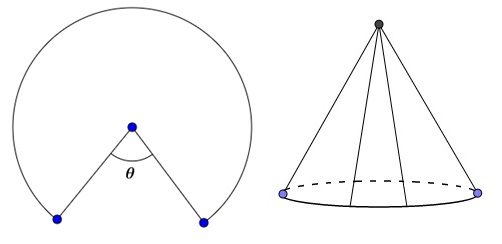Calculus Level 4A Cone is made from a circular sheet by cutting out a sector and gluing the cut edges of the remaining sheet together, as shown in the above figure. $\theta$ is the angle of the sector cut out for which the volume of the cone is maximized.

If the value of $\theta$ can be written as $a\left(1-\frac { \sqrt { b } }{ \sqrt { c } } \right)\pi$ , where $a,b,$ and $c$ are positive integers and $b$ and $c$ are not divisible by the square of any prime, determine the value of $a+b+c$.

×